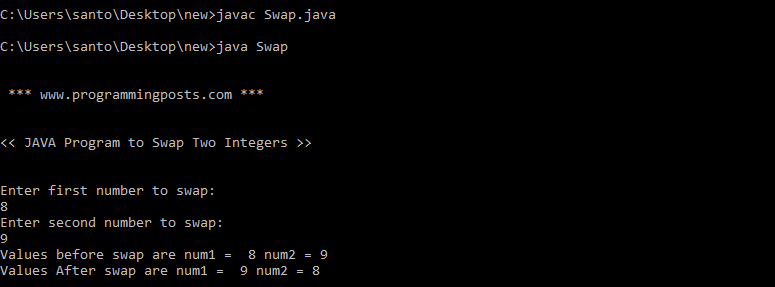# Java program to swap two variables

In computer programming, the act of swapping of two variables refers to mutually exchanging the values of the variables.

### Algorithm:

Step 1: START.

Step 2: Declare a, b, temp.

Step 3:Copy value of a to temp.

Step 4: Copy value of b to a.

Step 5: Copy value of temp to b.

Step 6: STOP.

### Source code:

```import java.util.Scanner;
class Swap
{
public static void main(String args[])
{
System.out.println("\n \n *** www.programmingposts.com *** \n \n");
System.out.println("<< JAVA Program to Swap Two Integers >> \n \n");
Scanner scanner = new Scanner(System.in);
System.out.println("Enter first number to swap:");
int num1 = scanner.nextInt();
System.out.println("Enter second number to swap:");
int num2 = scanner.nextInt();
System.out.println("Values before swap are num1 =  " + num1 + " num2 = " +num2);
int temp;
temp = num1;
num1 = num2;
num2 = temp;
System.out.println("Values After swap are num1 =  " + num1 + " num2 = " +num2);
}
}```

### Explanation:

In the above program, after user entering the two variables, the value of first variable is assigned to temporary variable. the value of second variable is assigned to the first variable. the value of temporary variable is assigned to second variable.

### Output:

C:\Users\santo\Desktop\new>javac Swap.java

C:\Users\santo\Desktop\new>java Swap

*** www.programmingposts.com ***

<< JAVA Program to Swap Two Integers  >>

Enter first number to swap:

8

Enter second number to swap:

9

Values before swap are num1 = 8 num2 = 9

Values After swap are num1 = 9 num2 = 8

### Screenshot of output:Screenshot of output